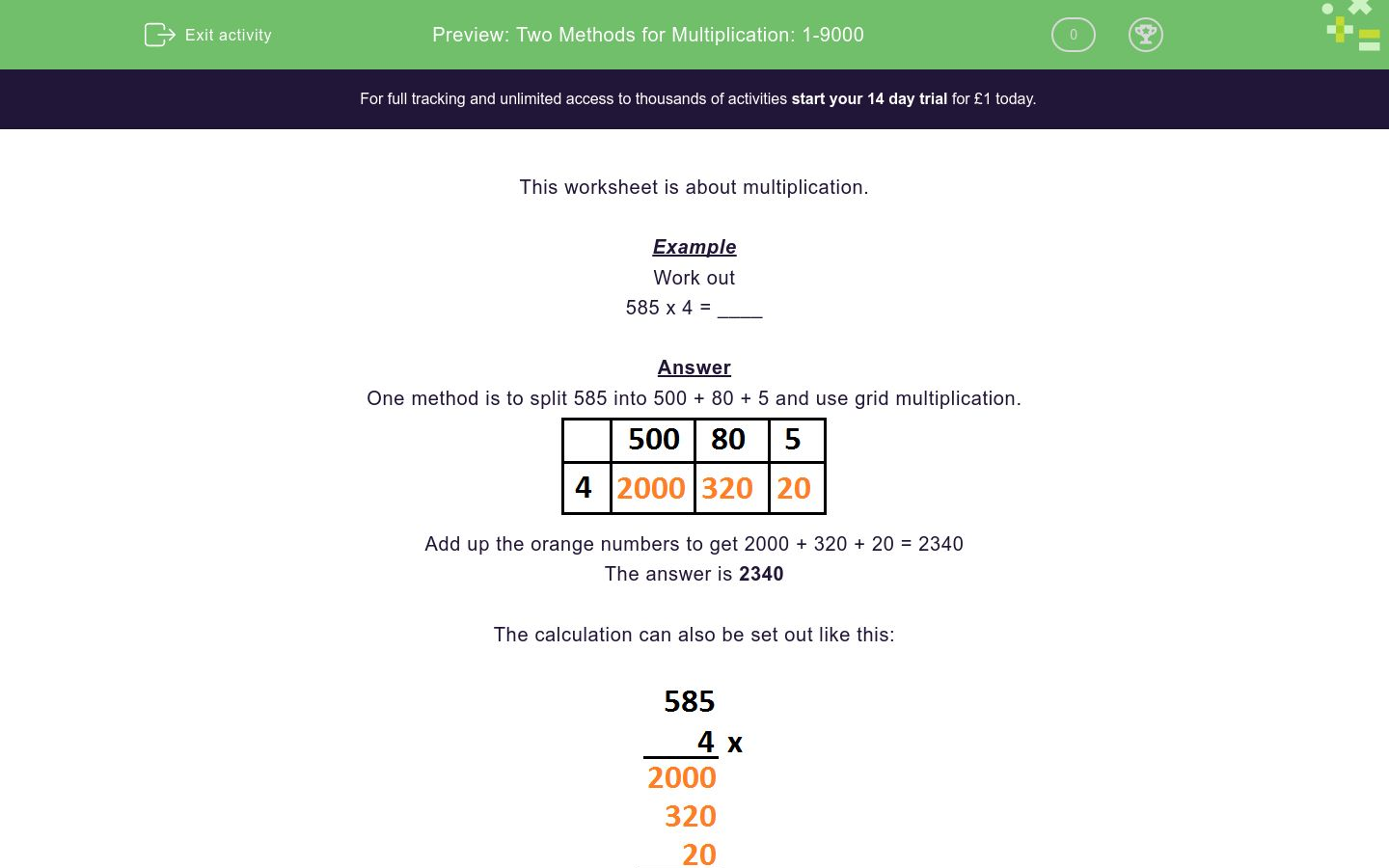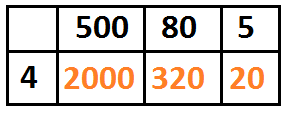# Two Methods for Multiplication: 1-9000

In this worksheet, students use two methods to complete multiplication problems.Key stage:  KS 2

Curriculum topic:   Number: Multiplication and Division

Curriculum subtopic:   Solve Multiplication/Division Problems

Difficulty level:### QUESTION 1 of 10

Example

Work out

585 x 4 = ____

One method is to split 585 into 500 + 80 + 5 and use grid multiplication.Add up the orange numbers to get 2000 + 320 + 20 = 2340

The calculation can also be set out like this:Work out

562 x 8 = ____

Work out

267 x 8 = ____

Work out

149 x 3 = ____

Work out

374 x 2 = ____

Work out

834 x 3 = ____

Work out

912 x 2 = ____

Work out

981 x 4 = ____

Work out

749 x 8 = ____

Work out

396 x 8 = ____

Work out

381 x 9 = ____

• Question 1

Work out

562 x 8 = ____

4496
EDDIE SAYS
Step 1. Multiply 500 x 8 = 4000 Step 2. Multiply 60 x 8 = 480 Step 3. Multiply 2 x 8 = 16 Step 4. Add 4000 + 480 + 16 = 4496
• Question 2

Work out

267 x 8 = ____

2136
EDDIE SAYS
Step 1. Multiply 200 x 8 = 1600 Step 2. Multiply 60 x 8 = 480 Step 3. Multiply 7 x 8 = 56 Step 4. Add 1600 + 480 + 56 = 2136
• Question 3

Work out

149 x 3 = ____

447
EDDIE SAYS
Step 1. Multiply 100 x 3 = 300 Step 2. Multiply 40 x 3 = 120 Step 3. Multiply 9 x 3 = 27 Step 4. Add 300 + 120 + 27 = 447
• Question 4

Work out

374 x 2 = ____

748
EDDIE SAYS
Step 1. Multiply 300 x 2 = 600 Step 2. Multiply 70 x 2 = 140 Step 3. Multiply 4 x 2 = 8 Step 4. Add 600 + 140 + 8 = 748
• Question 5

Work out

834 x 3 = ____

2502
EDDIE SAYS
Step 1. Multiply 800 x 3 = 2400 Step 2. Multiply 30 x 3 = 90 Step 3. Multiply 4 x 3 = 12 Step 4. Add 2400 + 90 + 12 = 2502
• Question 6

Work out

912 x 2 = ____

1824
EDDIE SAYS
Step 1. Multiply 900 x 2 = 1800 Step 2. Multiply 10 x 2 = 20 Step 3. Multiply 2 x 2 = 4 Step 4. Add 1800 + 20 + 4 = 1824
• Question 7

Work out

981 x 4 = ____

3924
EDDIE SAYS
Step 1. Multiply 900 x 4 = 3600 Step 2. Multiply 80 x 4 = 320 Step 3. Multiply 1 x 4 = 4 Step 4. Add 3600 + 320 + 4 = 3924
• Question 8

Work out

749 x 8 = ____

5992
EDDIE SAYS
Step 1. Multiply 700 x 8 = 5600 Step 2. Multiply 40 x 8 = 320 Step 3. Multiply 9 x 8 = 72 Step 4. Add 5600 + 320 + 72 = 5992
• Question 9

Work out

396 x 8 = ____

3168
EDDIE SAYS
Step 1. Multiply 300 x 8 = 2400 Step 2. Multiply 90 x 8 = 720 Step 3. Multiply 6 x 8 = 48 Step 4. Add 2400 + 720 + 48 = 3168
• Question 10

Work out

381 x 9 = ____

3429
EDDIE SAYS
Step 1. Multiply 300 x 9 = 2700 Step 2. Multiply 80 x 9 = 720 Step 3. Multiply 1 x 9 = 9 Step 4. Add 2700 + 720 + 9 = 3429
---- OR ----

Sign up for a £1 trial so you can track and measure your child's progress on this activity.

### What is EdPlace?

We're your National Curriculum aligned online education content provider helping each child succeed in English, maths and science from year 1 to GCSE. With an EdPlace account you’ll be able to track and measure progress, helping each child achieve their best. We build confidence and attainment by personalising each child’s learning at a level that suits them.

Get started